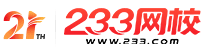#计算机二级

•扫描二维码进入

• 题 库扫描二维码进入

• 移动233网校移动

• 移动APP扫描二维码下载APP

## 2019年3月计算机二级MySQL基础试题及答案(13)

1.设int x=7，则～x的值是( )。

A.-8

B.7

C.-1

D.1

2.有以下程序：

void fun(char*a，char*b)

{ a=b; (*a)++; }

main()

{ char c1='A '，c2='a'，*p1，*p2;

p1=&c1;p2=*c2;fun(p1，p2);

printf("%C%c\n"，c1，c2);

}

A.Ab

B.aa

C.Aa

D.Bb

3.有以下程序：

int fun(int n)

{ if(n==l)return l;

else return(n+fun(n一1))：

}

main()

{ int x;

scanf("%d"，&x);x=fun(x);printf("%d\n"，

x)：

}

A.55

B.54

C.65

D.45

4.有以下程序：

void f(int x，int y)

{ int t;

if(x<Y){T=X;X=Y;Y=T;)< p>

}

main()

{ int a=4，b=3，c=5;

f(a，b);f(a，c);f(b，c);

printf("%d，%d，%d\n"，a，b，c);

}

A.3，4，5

B.5，3，4

C.5，4，3

D.4，3，5

5.以下程序的输出结果是( )。

main()

{ char*s="12134211";

int v=(0，0，0，0)，k，i;

for(k=0;s[k];k++)

{ switch(s[k])

{ case'1'：i=0;

case'2'：i=1;

case'3'：i=2;

case'4'：i=3;}

}

v[i]++：

}

for(k=0;k<4;k++)printf("%d"，v[k]);

A.4 2 1 1

B.0 0 0 8

C.4 6 7 8

D.8 8 8 8

6.有以下程序：

main()

{ int x=102，y=012;

printf("%2d，%2d\n"，x，y);

}

A.10，01

B.02，12

C.102，10

D.02，10

7.有以下程序：

main()

{ int x=0，y=5，z=3;

while(z-->0&&++x<5)y=y-l;

printf("%d，%d，%d\n"，x，y，z);

}

A.3，2，0

B.3。2. 1

C.4，3，-1

D.5，2.-5

8.有以下程序：

main()

{ int i，n=0：

for(i=2;i<5;i++)

{ do

{ if(i%3) continue：

n++：

}while(!i);

n++：

}

printf("n=%d\n"，n);

}

A.n=5

B.n=2

C.n=3

D.n=4

9.下面程序的功能是输出以下形式的金字塔图案：main()

{ int i，j; *

for(i=l;i<-4;i++) ***

{ for(j=1;j<=4-i;j++) printf("");

*****

for(j=1;j<=____;j++)printf("

*"); *******

printf("\n");

}

}

A.i

B.2*i一1

C.n=2*i+1

D.i+2

10.函数ftell(fp)的作用是( )。

A.得到fp所指向文件的当前读写位置

B.初始化流式文件的位置指针

C.移动流式文件的位置指针

D.以上答案均正确\

• 海量免费模拟试题，在线测试。

• 各科每年真题试卷，实战演练！

• 逐章逐节针对练习，专项突破。

• 每天10道最新试题，随机练习。

• QQ群号：806931213

扫描二维码加群• ##### 微信订阅号：kszx233

扫描二维码加群